Courses

# Interconnected DC Generators Electrical Engineering (EE) Notes | EduRev

## Electrical Engineering (EE) : Interconnected DC Generators Electrical Engineering (EE) Notes | EduRev

The document Interconnected DC Generators Electrical Engineering (EE) Notes | EduRev is a part of the Electrical Engineering (EE) Course Electrical Machines.
All you need of Electrical Engineering (EE) at this link: Electrical Engineering (EE)

Interconnected DC Generators
In the last lecture, we have seen how to find out different currents and voltages of a compound generator when it is adjusted it is designed and adjusted for a particular terminal characteristics, it will be also interesting to find out how many series turns we will need in order to get that kind of a characteristics. Let us try to solve a problem like that for that let us assume.

(Refer Slide Time: 00:51)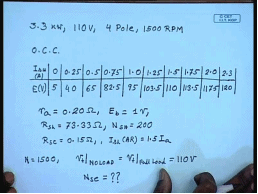We have a again a long shunt cumulative DC generator the following with the following rating 3.3 kilowatt, 110 volts, 4 pole, 1500 RPM. The O C C of this generator is given by the following table. The shunt field current and the induced voltage, the residual voltage is 5 volts for 0 point. This is in amperes and this is in volts. 0.25 40, 0.5 65, 0.75 82.5, 1.0 95 volts, 1.25 amperes 103.5 volts, 1.5 amperes 110 volts, 1.75 amperes 113.5 volts, 2.0 117.5 and 2.3 amperes gives you 120 volts on the whole for this machine.

Further the following data is given, armature resistance equal to 0.20 ohm. The total brush contact drop on two brushes equal to 1 volt, the shunt field resistance R s h equal to 73.33 ohm. Number of shunt field turn N s h equal to 200. The series field resistance equal to 15 ohm. The effective armature reaction field current that is I s h armature reaction is equal given is proportional to armature current and is given by 1.5 times I a.

So, if I want let us say at 15 N equal to 1500, we want the terminal voltage at no load should be equal to terminal voltage at full load equal to rated terminal voltage that is 110 volts. So, we want a flat compounding characteristics the question is what should be the number of series field turns. Let us see how to solve this problem for that.

(Refer Slide Time: 05:59)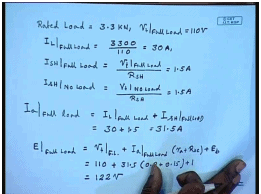We see that a weighted load is equal to 3.3 kilowatt, V t at full load equal to 110 volts. Therefore, I L at full load equal to 3300 divided by 110 this is equal to 30 amperes. So, I s h at full load since this is a long shunt connection I s h is V t at full load, long shunt connection field shunt field is connected across the load terminal and divided by R s h this comes to 1.5 amperes.

Similarly, I s h at no load is also V t at no load which is again 110 volts. This is also equal to 1.5 amperes. So, at full load what is the armature current I a at full load equal to I L at full load plus I s h at full load. This is equal to 30 ampere plus 1.5 equal to 31.5 ampere. So, what is the induced voltage E V. E at full load this is equal to terminal voltage at full load plus I a full load, and this is a long shunt connection therefore, this should be equal to r a plus R s e plus the brush contact drop this is equal to 110 plus 31.5 into 0.2 plus 0.15 plus 1 this comes to 122 volts.

(Refer Slide Time: 09:58)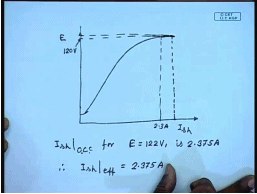The O C C data is given here from which we can plot the O C C of this. The O C C data gives for a maximum it gives up to 2.3 amperes and the corresponding voltage is 120 volts; however, we find that here the induced voltage will be 122 volts. So, it should be somewhat out of this range and you will have to extend the O C C; however, you do the exercise and you can find out the required field current comes out to be. So, I s h from O C C for E equal to 122 volts this comes to is 2.375 amperes. Now it is mentioned that the. So, in order to generate a induced voltage of 122 volt for this compound generator. The I s h effective should be I s h effective should be 2.375 amperes.

(Refer Slide Time: 12:23)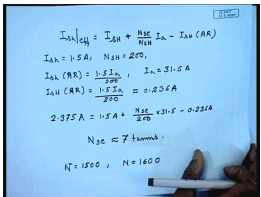But for long shunt for long shunt connection we know I s h heffective equal to I s h plus N s e by N s h into I a minus I s h armature reaction. Now we know I S H equal to 1.5 amperes. N s h equal to 200, and also we know I s h for armature reaction the effective armature reaction and field current equal to 1.5 I a at full load I a equal to 31. 5 amperes. So, I s h into armature reaction at full load equal to 1.5 by 200 I a by 200 that is the shunt field current 0.236 amperes.

And we know that I s h effective is. So, we have 2.375 amperes equal to 1.5 ampere plus N s e by N s h is 200 into 31.5 minus 0.236 ampere. Solving this we get N s E approximately equal to 7 turns. Now this will give a flat compounding characteristics at a rotational speed of 1500 R p m it will be interesting to see how the characteristics will change if the rotational speed let us say increases from N equal to 1500 to N equal to 1600. Let us first see what will happen to the no load voltage.

(Refer Slide Time: 16:00)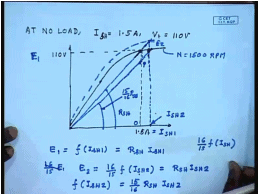At no load with the connection we know that, at no load I f equal to I s h equal to 1.5 amperes, and the terminal voltage V t equal to 110 volts. So, let us find out what will happen at 1600 R P M for that first draw the O C C at 1500 R P M, and so this is the 1.5 ampere, and the corresponding voltage is 110 volts. We can of course so, this is the field load line. We can of course draw the this is at N equal to 1500 R P M. We can similarly, draw the O C C at 1600 R P M and find out the intersection point from that curve, but as you’ll find that there is no need to draw this O C C. In fact, the field current can be very easily computed by finding out this point p what should be this point p let us say this I f 1 is equal to some current I S H 1.

So, from the and let this be E 1. So, E 1 equal to the magnetising function characteristics f into of I S H 1 at 1500 R P M . So, at 1600 R P M this is also equal to R S H I S H 1. At 1600 R P M this will become 16 by 15 times E 1. So, at 1600 R P M the O C C will be given by 16 by 15 into f of I S H. So, let us say it will intersect at a this intersection point is given by I S H 2 and the corresponding voltage is E 2. So, E 2 equal to this is E 2 E 2 equal to 16 by 15 f of I S H 2. This should be equal to R S H into I S H 2. Hence the intersection the current I S H can be found out from f of I S H 2 equal to 15 by 16 time R S H into I S H 2. That is if this is with a slope of R S H we simply draw a new field load line with a slope of 15 by 16 R S H. And wherever it intersects with the O C C at R P M we will give the value of I S H 2 following this procedure.

(Refer Slide Time: 21:35)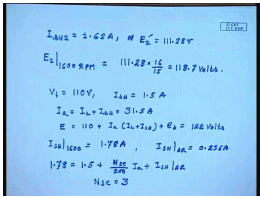We find that I S H 2 will be, for this case will be 1.62 amperes and the corresponding voltage E 2 dash that is this voltage E 2 dash will be equal to 111.28 volt. So, at 16 also. So, E 2 that is the no load terminal voltage at 1600 R P M will be 111.28 into 16 by 15 that is 118.7 volts. Now, if we want the same 110 volt at 1600 R p m how many series turns we will need. So, on full load V t equal to we want to be same 110 volts then the I S H will be equal to 1.5 amperes and I a will again be I L plus I S H equal to 31.5 amperes, and E will again be equal to 110 plus I a into I L plus I S H plus E b this will again be 122 volts. Now in the same manner as we found out from the for the no load condition we know that in order to generate this voltage I S H at 1600 R p m will be effective I S H at 1600 R p m should be 1.78 amperes.

We know I S H A R at full load equal to 0.236 ampere. Therefore effective I S H 1.78 should be equal to actual I S H plus N s e by 200 I a plus I S H A R which gives that the if we now need to skip it at 110 volts required N s e will be just 3. So, the operating speed has a very prominent effect on the output characteristics of a compound generator; however, by adjusting the number of shunt field turns we can get the desired characteristics. Now we have seen that any electrical equipment has a particular output rating the same is true for DC generators, it is designed to supply certain load.

(Refer Slide Time: 26:00)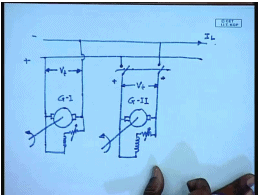Now, if the load increases then what do I do, in which case one approach is to interconnect different DC generators assumed. Suppose you had a already had a DC generator a shunt generator let us say, which was supplying a load of I L, if I L is less than the rated current of this DC generator then only this generator will be able to supply this, but if there is a possibility that this I L can be larger than the connected generator then it will be imperative to bring in another generator and connect them in parallel for that initially this generator will first be run at no load again we are assuming this is another shunt generator. Before this generator is connected it must be ensured that the terminal voltage. Let us say it was supplying current of I L at a terminal voltage of V t.

It must be made sure that the incoming generator at no load generates a terminal voltage of V t. Then this switch can be closed and once the switch is closed then these two generators are connected in parallel; however, that does not ensure that the second generator shares any load. In fact, if the no load voltage of the incoming generator has been adjusted to match the terminal voltage of the existing generator when it is just connected the second generator will not share any load current.

(Refer Slide Time: 30:01)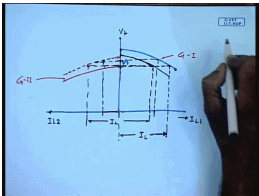That can be seen from the output characteristics of these two generators. Let us say this is a shunt generator. So, initially this was the output characteristics of this generator. It was supplying this is the load current of the first generator, this is the load current of the second generator. Let us say this was the terminal voltage, and it was supplying the full current I L. Now the second for the second generator we have adjusted and the terminal voltage was V t. This was V t we have adjusted the no load voltage of the second generator to be V t. So, this is the characteristics of the second generator. Now of course, during this period the second generator load current is 0, the first generator load current I L 1 is the total load current I L.

Now, if I want to transfers some of the load current to the second generator this is generator I and this is generator II, its field excitation has to be increased. So, that the output characteristics moves upward let us say by some increase we bring the second generator characteristics as per the dotted line. If the load remains constant then what will happen total load current will remain constant. So, some load will be shared by the second generator, so that the total load current still remain. Now this will be the load shared by the second generator, and this will be the load shared by the first generator; however, we see that if we do this then there is a increase in the terminal voltage.

If we want to bring the terminal voltage back to the original level then the field excitation of the first generator must now be reduced. So, that now this becomes this will once the field excitation of the first generator is reduced then its output characteristics will move down this will reduce the load shared by the first generator and that load will be transferred to the second generator, thus for a for interconnected DC shunt generators it is possible to change the load shared by these generators by controlling their field excitation; however, the situation is somewhat more complicated if a compound generator is used instead.

(Refer Slide Time: 34:53)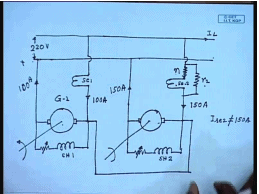Let us see the situation of the compound generator. This is the DC bus to which these two generators are connected. To this or let us say short shunt generators this is generator 1, this is s e field 1. Similarly, in the second generator if its own this is the shunt field 1, this is shunt field 2, this is series field 2. Now let us see what will happen suppose these two generators were sharing the total load current I L in some manner suddenly suppose for some reason the field excitation of this generator increases then what will happen, the terminal voltage of this generator will move up hence the share of the load current of this generator will increase.

As the share increases the series field excitation also increases as the series field excitation increases the induced voltage increases hence this further increases the load current of this is a positive feedback mechanism particularly, if it is a over compounded generator. In which case then even a small change in the field excitation of one of the generators will create a very large disturbance or inequality in the sharing current sharing pattern of the parallel connected generators. Therefore, if we want to use compound generators in parallel simply this connection is not good enough we have to make a special connection called the equalizer bar.

So, in effect what we are doing when connecting the machines not only parallel at the terminal, but also parallel at the armature point. So, if we do this then the armature of the two generators are in parallel. The series field of the two generators in parallel. So, any increase in the armature current of the of one of the generators is shared by both the series fields. And in fact, this sharing can be controlled by connecting additional resistances across one of the generators this is R 1, this is R 2, and suitably designing them and thus preventing this positive feedback mechanism it is possible to find out to calculate the values of this resistances and we will explain this with the help of a example. Let us say we have two over compounded short shunt generators connected in this manner with the following data.

(Refer Slide Time: 40:37)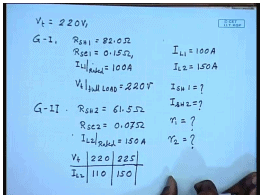The rated bus voltage V t equal to 220 volts, for generator 1, R S H 1 equal to 82. 0 ohm R s e 1 equal to 0.15 ohm, I L 1 at full load I L 1 rated equal to 100 amperes, and from load characteristics we know for V t at 100 ampere, V t full load for this machine is 220 volts similarly, for the second machine generator 2, R S H 2 equal to 61.5 ohm, R s e 2 equal to 0.07 ohm, I L 2 rated equal to 150 amperes.

And from the load characteristics we know V t, I L 2 at 110 amperes of load current, the V t is 220 volts and at 150 amperes of rated current this 225 volt that is this generator is over compounded. We want both the generators to be to operate at the rated load current that is we want I L 1 to be equal to 100 amperes, and I L 2 to be equal to 150 ampere. So, what should be the values of I S H 1, I S H 2 and what should be the value of R 1 and R 2, where this is how R 1 and R 2 this is called a diverted resistance this is called a series resistance. R 1 equal to how much R 2 equal to how much.

We are supposed to find this out for this we note that both the machines are supplying their rated current. This voltages is 220 volt this is positive. So, this is giving a current of 100 ampere which is its rated current similarly, this is giving a current of 150 ampere. Note that the current this is 150 ampere, but the series field current I s E 2is not 150 ampere while I s e 1 is this is 100 amperes, this current flowing through I s E 2.

(Refer Slide Time: 45:34)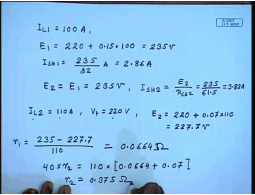Can be adjusted by controlling the diverted resistance R 2, since I L one is equal to 100 ampere in the induced voltage E 1 should be equal to terminal voltage 220 volts plus this is short shunt connection. So, this is the shunt field resistance into load current hundred this comes to 235 volts. Hence this is short shunt connections. So, I S H 1 should be equal to 235 divided by the R S H 1 which is 82 this comes to 2.86 amperes. And this voltage is E 1 this is E 1. Now because of this equalizer connection see this end is connected here and this end is connected here. So, E 1 must be equal to E 2. So, because of the equalizer connection E 2 equal to E 1 equal to 235 volts, and the shunt field of the second machine is also it is a short shunt. So, you connected across E 2. So, I S H 2 this will be equal to 3.82 amperes.

Now, let us see we have from the load data you have seen when the machine 1 supplies 100 ampere of load current, its terminal voltage is 220 volt which is same as the bus voltage; however, for the machine 2, the data shows that when I L 2 is when V t is 220 volts then I L 2 is 110 amperes. So, if 110 amperes flows then if I L 2 is 110 amperes then V t equal to 220 volts. The armature terminal voltage armature terminal voltage if I L 2 is to be 110 ampere then in which case E 2 becomes 220 plus at a terminal voltage of 220 I L 2 is 110 volt amperes.

So, this will become 220 plus 0.07 into 110 equal to 227.7 volts, but since these are parallel connected E 2 must be equal to 235 volts. So, the additional voltage of... So, this voltage of 235 minus 227.7 volt, this additional voltage must be dropped across this resistance R 1. So, R 1 should be equal to this divided by 110 amperes because such series field winding current must be equal to 110 ampere, so as to generate 220 volt of in 220 volt across the 220 volt terminal voltage from machine 2. So, this gives the value of R 1.

So, this R 1 comes to 0.0664 ohm then what should be R 2 if the current the current through the series field is 110 amperes therefore, the current through since the total current is 100 amperes this is 40 ampere. So, 40 into R 2 these are connected in parallel equal to 110 into 0.06654 plus the shunt field to resistance which is 0.07 ohm. So, from here we get R 2 to be equal to 0.375 ohms. So, we say by this method we can find out what should be the value of R 1 and R 2.

Thank you.

Offer running on EduRev: Apply code STAYHOME200 to get INR 200 off on our premium plan EduRev Infinity!

61 docs|20 tests

,

,

,

,

,

,

,

,

,

,

,

,

,

,

,

,

,

,

,

,

,

;